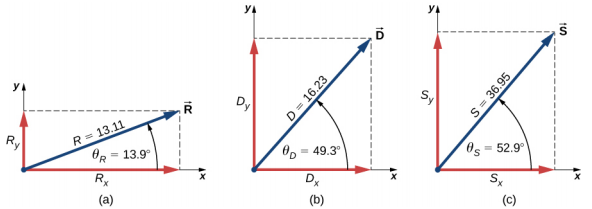$$\require{cancel}$$

# 2.7: Algebra of Vectors Examples

•• Contributed by OpenStax
• General Physics at OpenStax CNXFigure 2.26, the jogger’s displacement vector on the top of the hill is $$\vec{D}_{RB}$$ = (−50.0 m) $$\hat{i}$$. His net displacement vector is

$$\vec{D}_{AB} = \vec{D}_{AT} + \vec{D}_{TB} \ldotp$$

Therefore, his displacement vector $$\vec{D}_{TB}$$ along the stairs is

$$\begin{split} \vec{D}_{AT}& = \vec{D}_{AB} - \vec{D}_{TB} = (-90.0 \hat{i} + 30.0 \hat{j})m - (-50.0 m)\hat{i}\) = [(-90.0 50.0) hat{i} + 30.0 \hat{j})]m \\ & = (-40.0 \hat{i} + 30.0 \hat{j})m \ldotp \end{split}$$

Its scalar components are DATx = −40.0 m and DATy = 30.0 m. Therefore, we must have

$$200w = |− 40.0|m and 200h = 30.0 m \ldotp$$

Hence, the step width is w = $$\frac{40.0\; m}{200}$$ = 0.2 m = 20 cm, and the step height is w = $$\frac{30.0\; m}{200}$$ = 0.15 m = 15 cm. The distance that the jogger covers along the stairs is

$$\vec{D}_{AT} = \sqrt{\vec{D}_{ATx}^{2} + \vec{D}_{ATy}^{2}} = \sqrt{(-40.0)^{2} + (30.0)^{2}}m = 50.0\; m \ldotp$$

Thus, the actual distance he runs is DAT + DTB = 50.0 m + 50.0 m = 100.0 m. When he makes a loop and comes back from the fountain to his initial position at point A, the total distance he covers is twice this distance,or 200.0 m. However, his net displacement vector is zero, because when his final position is the same as his initial position, the scalar components of his net displacement vector are zero (Equation 2.13).

Example 2.14

# The Unit Vector of Direction

If the velocity vector of the military convoy in Example 2.8 is $$\vec{v}$$ = (4.000 $$\hat{i}$$ + 3.000 $$\hat{j}$$ + 0.100 $$\hat{k}$$)km/h, what is the unit vector of its direction of motion?

# Strategy

The unit vector of the convoy’s direction of motion is the unit vector $$\hat{v}$$ that is parallel to the velocity vector. The unit vector is obtained by dividing a vector by its magnitude, in accordance with Equation 2.26.

# Solution

The magnitude of the vector $$\vec{v}$$ is

$$v = \sqrt{v_{x}^{2} + v_{y}^{2} + v_{z}^{2}} = \sqrt{4.000^{2} + 3.000^{2} + 0.100^{2}}km/h = 5.001\; km/h \ldotp$$

To obtain the unit vector $$\hat{v}$$, divide $$\vec{v}$$ by its magnitude:

$$\begin{split} \hat{v}& = \frac{\vec{v}}{v} = \frac{(4.000 \hat{i} + 3.00 \hat{j} + 0.100 \hat{k})km/h}{5.001\; km/h} \\ & = \frac{(4.000 \hat{i} + 3.000 \hat{j} + 0.1100 \hat{k})}{5.001} \\ & = \frac{4.000}{5.001} \hat{i} + \frac{3.000}{5.001} \hat{j} + \frac{0.100}{5.001} \hat{k} \\ & = (79.98 \hat{i} + 59.99 \hat{j} + 2.00 \hat{k}) \times 10^{-2} \ldotp \end{split}$$

# Significance

Note that when using the analytical method with a calculator, it is advisable to carry out your calculations to at least three decimal places and then round off the final answer to the required number of significant figures, which is the way we performed calculations in this example. If you round off your partial answer too early, you risk your final answer having a huge numerical error, and it may be far off from the exact answer or from a value measured in an experiment.

Exercise 2.10

Verify that vector $$\hat{v}$$ obtained in Example 2.14 is indeed a unit vector by computing its magnitude. If the convoy in Example 2.8 was moving across a desert flatland—that is, if the third component of its velocity was zero—what is the unit vector of its direction of motion? Which geographic direction does it represent?

Contributors

• Samuel J. Ling (Truman State University), Jeff Sanny (Loyola Marymount University), and Bill Moebs with many contributing authors. This work is licensed by OpenStax University Physics under a Creative Commons Attribution License (by 4.0).